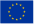next
previous
itemsFigure

# Overall changes in energy-related emissions by main group of air pollutants in the EU-25, 1990-2003

Figure Created 12 Nov 2009 Published 12 Nov 2009 Last modified 29 Nov 2012
Topics:
The emissions of acidifying pollutants (SO2, NOX and NH3) are each weighted by an acid equivalency factor prior to aggregation to represent their respective acidification potentials

These factors are: w(SO2) = 2/64 acid eq/g = 31.25 acid eq/kg, w(NOX) = 1/46 acid eq/g = 21.74 acid eq/kg and w(NH3) = 1/17 acid eq/g = 58.82 acid eq/kg. For tropospheric ozone formation, the relative impact of the combined contribution of NOX, NMVOC, CO and CH4 can be assessed based on their tropospheric ozone forming potentials (TOFP). These are: 1.220, 1.000, 0.110 and 0.014, respectively. For particle formation, emissions are estimated using the following aerosol 'formation factors': primary PM10 = 1, NOX = 0.88, SO2 = 0.54 and NH3 = 0.64.